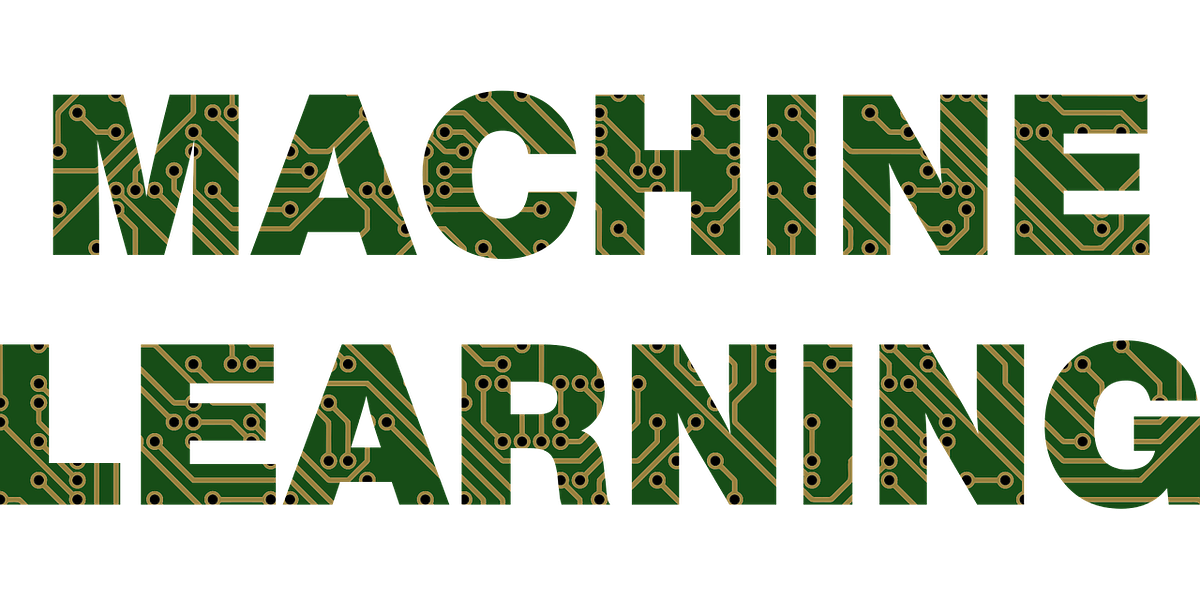# Multiple Linear Regression model using Python: Machine LearningMultiple Linear Regression model using Python: Machine Learning. Learning how to build a basic multiple linear regression model in machine learning using Jupyter notebook in python

Linear regression performs a regression task on a target variable based on independent variables in a given data. It is a machine learning algorithm and is often used to find the relationship between the target and independent variables.

TheSimple Linear Regressionmodel is to predict the target variable using one independent variable.

When one variable/column in a dataset is not sufficient to create a good model and make more accurate predictions, we’ll use a multiple linear regression model instead of a simple linear regression model.

The line equation for the multiple linear regression model is:

`y = β0 + β1X1 + β2X2 + β3X3 + .... + βpXp + e`

Before proceeding further on building the model using python, we need to consider some things:

1. Adding more variables isn’t always helpful because the model may ‘over-fit,’ and it’ll be too complicated. The trained model doesn’t generalize with the new data. It only works on the trained data.
2. All the variables/columns in the dataset may not be independent. This condition is called `**multicollinearity**`, where there is an association between predictor variables.
3. We have to select the appropriate variables to build the best model. This process of selecting variables is called `**Feature selection**`.

We’ll discuss points 2 & 3 using python code.

Now, let’s dive into the `Jupyter notebook` and see how we can build the Python model.

## Simple Linear Regression Model using Python: Machine Learning

Learning how to build a simple linear regression model in machine learning using Jupyter notebook in Python. This article will see how we can build a linear regression model using Python in the Jupyter notebook.

## A Deep Dive into Linear Regression

Lets begin our machine learning journey. A Deep Dive into Linear Regression. Why is this not learning? Because if you change the training data or environment even slightly, the algorithm will go haywire! Not how learning works in humans. If you learned to play a video game by looking straight at the screen, you would still be a good player if the screen is slightly tilted by someone, which would not be the case in ML algorithms.

## Linear Regression VS Logistic Regression (MACHINE LEARNING)

Linear Regression VS Logistic Regression (MACHINE LEARNING). Linear Regression and Logistic Regression are two algorithms of machine learning and these are mostly used in the data science field.

## Regression: Linear Regression

Machine learning algorithms are not your regular algorithms that we may be used to because they are often described by a combination of some complex statistics and mathematics.

## Towards Machine Learning — Linear Regression and Polynomial Regression

I decided to write few brief articles regarding this topic, which are intended to help people new to this topic dive in the interesting world of Machine Learning. Today we go further, and tackle Linear Regression, another extremely popular and wide used technique.